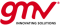If you wish to contribute or participate in the discussions about articles you are invited to contact the Editor

# AccuracyFundamentals
Title Accuracy
Edited by GMV
Level Basic
Year of Publication 2011The accuracy of an estimated or measured position of a craft (vehicle, aircraft, or vessel) at a given time is the degree of conformance of that position with the true position, velocity and/or time of the craft. Since accuracy is a statistical measure of performance, a statement of navigation system accuracy is meaningless unless it includes a statement of the uncertainty in position that applies[nb 1].

## Measuring Accuracy

Although being very easily understood from a conceptual point of view, the way that accuracy is measured and what is measured is not always obvious. The accuracy concept is generally used to measure the accuracy of positioning but can be also be used to measure the accuracy of velocity and even the accuracy of timing.

Navigation errors generally follow a known error distribution and the uncertainty in position can be expressed as the probability that the error will not exceed a certain amount. Further complications arise because some navigation systems are linear (one-dimensional) while others provide two or three dimensions of position.

For positioning there are 3 variants depending on the number of dimensions being considered: one-dimensional accuracy (used for vertical accuracy), bidimensional accuracy (used for horizontal accuracy) and tridimensional accuracy (combining horizontal and vertical accuracy).

In literature and in system/product specifications it can be found measurements of accuracy such as CEP, rms, Percentile 67%, Percentile 95%, 1 sigma, 2 sigma. Some of these accuracy measures are averages while others are counts of distribution:

• x Percentile (x% or x-th): Means that x% of the positions calculated have an error lower or equal to the accuracy value. Typical used values are 50%, 67%, 75% and 95%. Having an accuracy of 5m (95%) means that in 95% of the time the positioning error will be equal or below 5m.
• Circular Error Probable (CEP): Percentile 50%. Means that 50% of the positions returned calculated have an error lower or equal to the accuracy value.
• Root Mean Square Error (rms): The square root of the average of the squared error. This measurement is an average but assuming that the error follows a normal distribution (which is close but not exactly true) it will correspond to the percentile 68% in one-dimensional distributions (e.g. vertical error or timing error) and percentile 63% for bidimensional distributions (e.g. horizontal error). For the horizontal error this measurement is also referred as drms and can have variants such as 2rms or 2drms (2 times rms).
• x sigma: 1 sigma corresponds to one standard deviation and x sigma corresponds to x times 1 sigma. Assuming normal distributions 1 sigma corresponds to Percentile 68% in one-dimensional distributions and Percentile 39% for bidimensional distributions.

Less used that the previous measurements are the:

• Mean Error: Average error. Corresponds to Percentile 68% in one-dimensional distributions and to Percentile 54% for bidimensional distributions.
• Standard Deviation: Standard deviation of the error. Same as one sigma. Corresponds to Percentile 58% in one-dimensional distributions and to Percentile 39% for bidimensional distributions.

Although the mean error and standard deviation are less used as accuracy measurements, assuming normal distributions its use is as legitimate as the other measurements usually used.

## Relationship between Accuracy Measurements

Assuming normal distributions these accuracy measurements can be converted between themselves. There is a correspondence between sigmas and percentiles. This correspondence can be used to convert between accuracy measurements since an accuracy of 1m (1 sigma) corresponds to 2m (2 sigma) , 3m (3 sigma) and xm (x sigma).

For one-dimensional distributions:

Sigma Percentile
0,67 0,5 (CEP)
0,80 0,58 (mean error)
1 0,6827 (rms and std deviation)
1,15 0,75
1,96 0,95
2 0,9545
2,33 0,98
2,57 0,99
3 0,9973
4 0,999936
5 0,99999942
6 0,999999998

For bidimensional distributions (Rayleigh distribution) :

Sigma Percentile
1 0,394 (std deviation)
1,18 0,5 (CEP)
1,25 0,544 (mean error)
1,414 0,632 (rms)
1,67 0,75
2 0,865
2,45 0,95
2,818 0,982 (2rms)
3 0,989
3,03 0,99
4 0,9997
5 0,999997
6 0,999999985